Constructing angles - 60, 90, 45, 30 and others

Chapter 11 Class 9 Constructions
Concept wise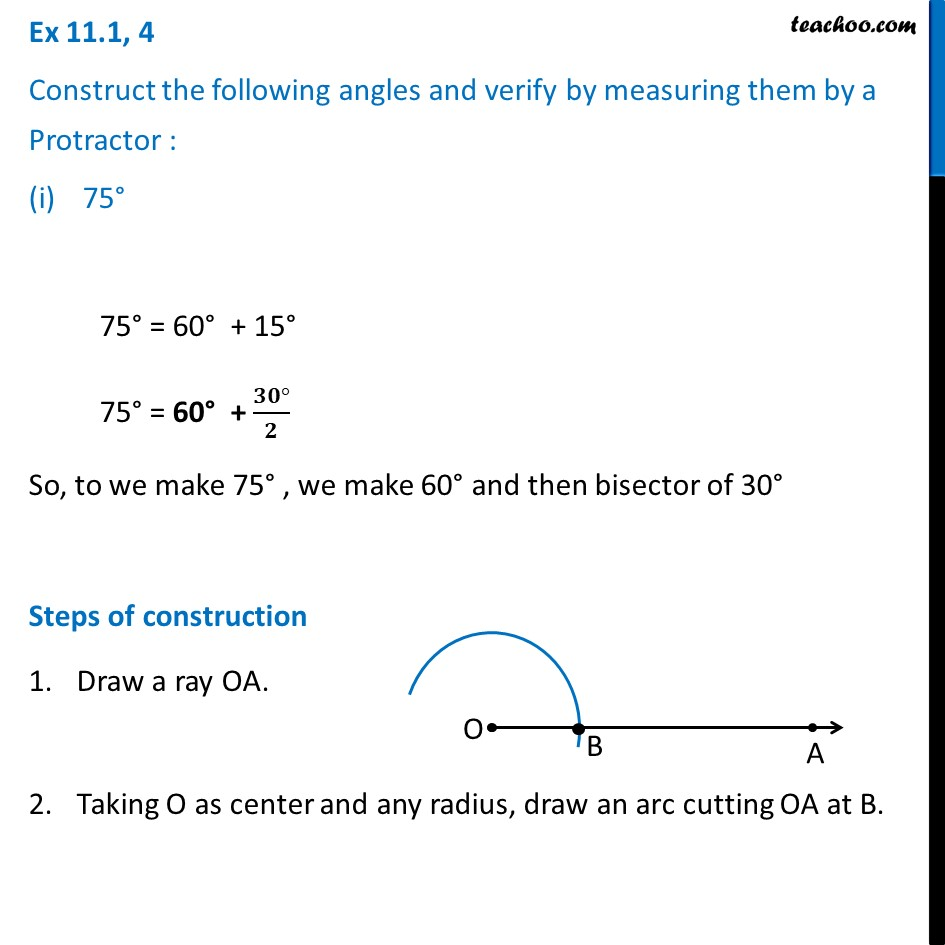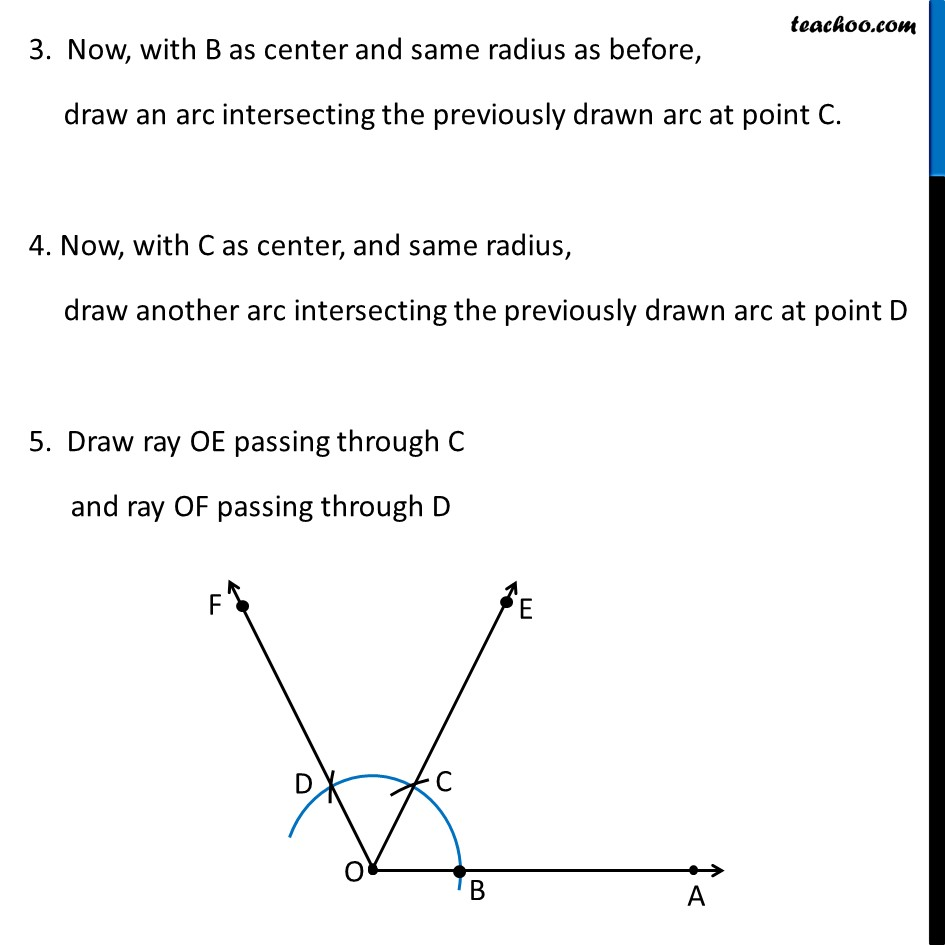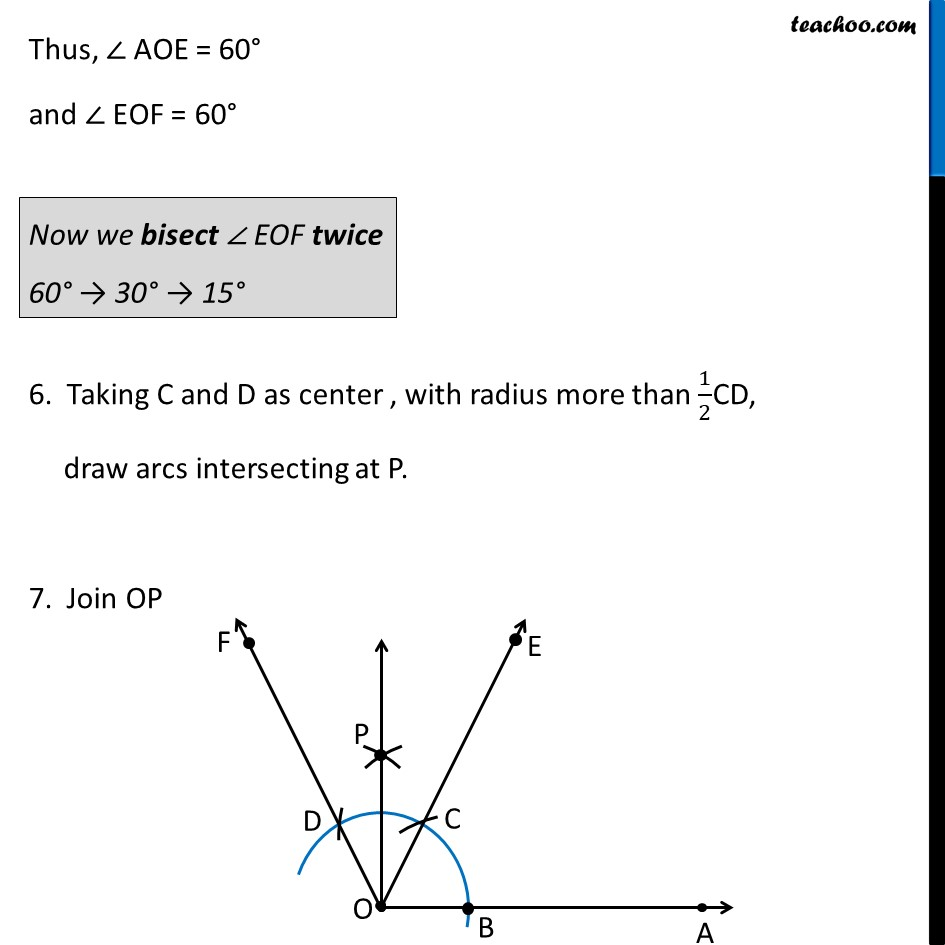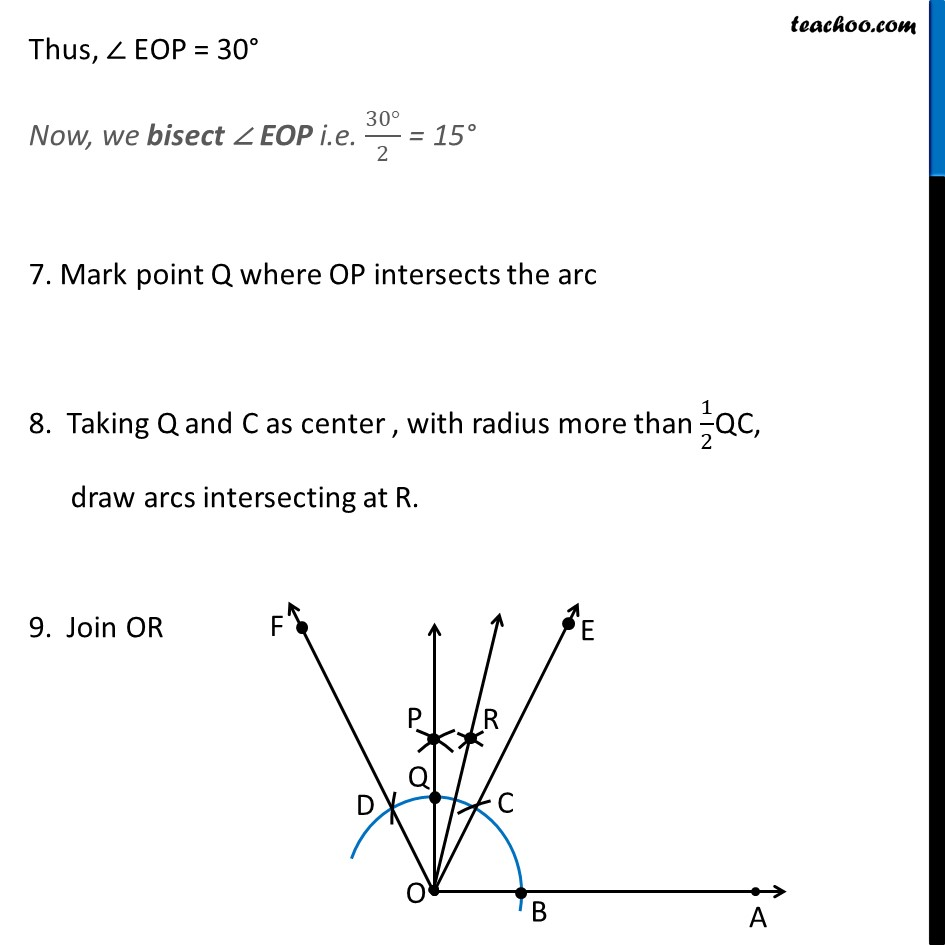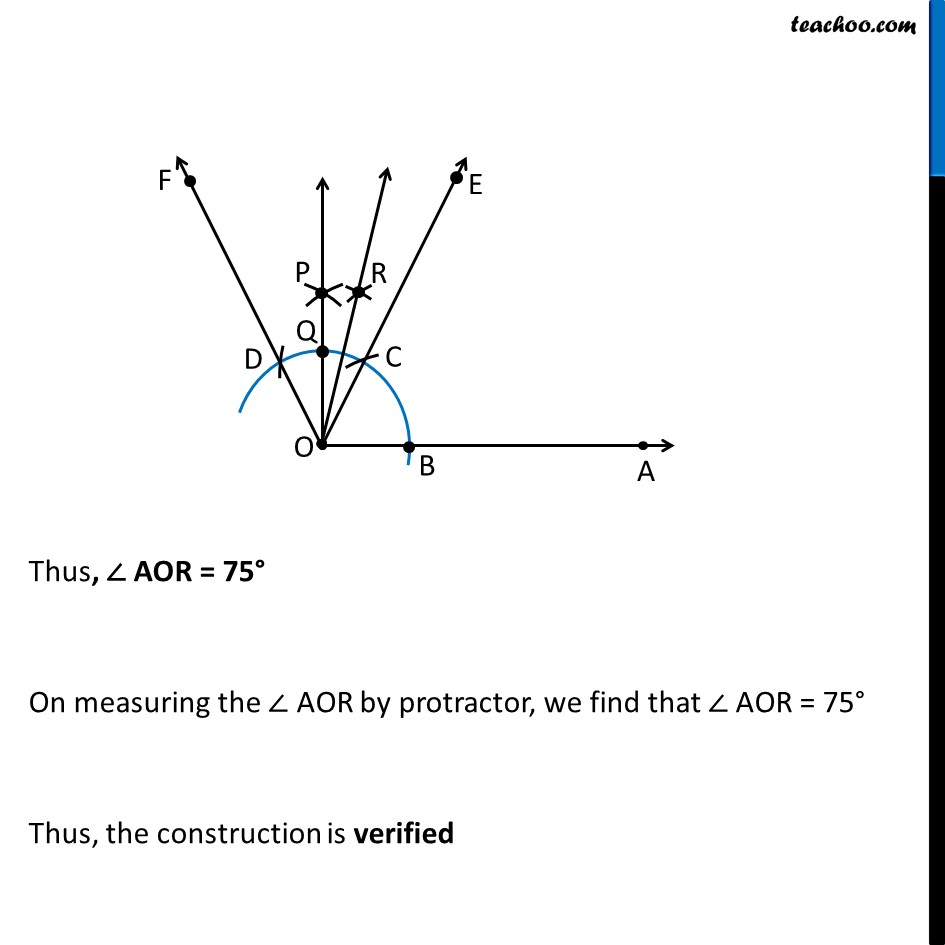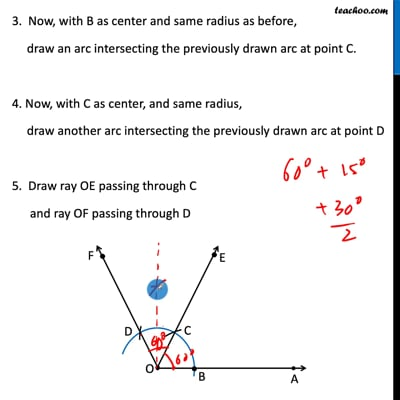This video is only available for Teachoo black users

Introducing your new favourite teacher - Teachoo Black, at only ₹83 per month

### Transcript

Ex 11.1, 4 Construct the following angles and verify by measuring them by a Protractor : 75° 75° = 60° + 15° 75° = 60° + (𝟑𝟎°)/𝟐 So, to we make 75° , we make 60° and then bisector of 30° Steps of construction Draw a ray OA. Taking O as center and any radius, draw an arc cutting OA at B. 3. Now, with B as center and same radius as before, draw an arc intersecting the previously drawn arc at point C. 4. Now, with C as center, and same radius, draw another arc intersecting the previously drawn arc at point D 5. Draw ray OE passing through C and ray OF passing through D Thus, ∠ AOE = 60° and ∠ EOF = 60° 6. Taking C and D as center , with radius more than 1/2CD, draw arcs intersecting at P. 7. Join OP Thus, ∠ AOE = 60° and ∠ EOF = 60° 6. Taking C and D as center , with radius more than 1/2CD, draw arcs intersecting at P. 7. Join OP Thus, ∠ EOP = 30° Now, we bisect ∠ EOP i.e. (30°)/2 = 15° 7. Mark point Q where OP intersects the arc 8. Taking Q and C as center , with radius more than 1/2QC, draw arcs intersecting at R. 9. Join OR Thus, ∠ AOR = 75° On measuring the ∠ AOR by protractor, we find that ∠ AOR = 75° Thus, the construction is verified